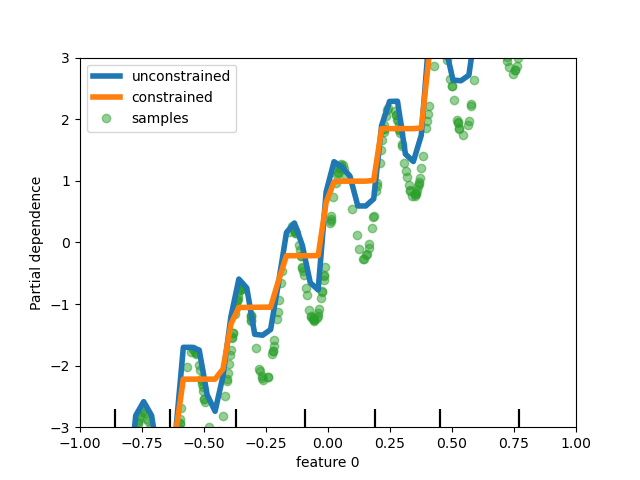# Release Highlights for scikit-learn 0.23¶

We are pleased to announce the release of scikit-learn 0.23! Many bug fixes and improvements were added, as well as some new key features. We detail below a few of the major features of this release. For an exhaustive list of all the changes, please refer to the release notes.

pip install --upgrade scikit-learn


or with conda:

conda install -c conda-forge scikit-learn


## Generalized Linear Models, and Poisson loss for gradient boosting¶

Long-awaited Generalized Linear Models with non-normal loss functions are now available. In particular, three new regressors were implemented: PoissonRegressor, GammaRegressor, and TweedieRegressor. The Poisson regressor can be used to model positive integer counts, or relative frequencies. Read more in the User Guide. Additionally, HistGradientBoostingRegressor supports a new ‘poisson’ loss as well.

import numpy as np
from sklearn.model_selection import train_test_split
from sklearn.linear_model import PoissonRegressor

n_samples, n_features = 1000, 20
rng = np.random.RandomState(0)
X = rng.randn(n_samples, n_features)
# positive integer target correlated with X[:, 5] with many zeros:
y = rng.poisson(lam=np.exp(X[:, 5]) / 2)
X_train, X_test, y_train, y_test = train_test_split(X, y, random_state=rng)
glm = PoissonRegressor()
glm.fit(X_train, y_train)
gbdt.fit(X_train, y_train)
print(glm.score(X_test, y_test))
print(gbdt.score(X_test, y_test))

0.35776189065725783
0.42425183539869404


## Rich visual representation of estimators¶

Estimators can now be visualized in notebooks by enabling the display='diagram' option. This is particularly useful to summarise the structure of pipelines and other composite estimators, with interactivity to provide detail. Click on the example image below to expand Pipeline elements. See Visualizing Composite Estimators for how you can use this feature.

from sklearn import set_config
from sklearn.pipeline import make_pipeline
from sklearn.preprocessing import OneHotEncoder, StandardScaler
from sklearn.impute import SimpleImputer
from sklearn.compose import make_column_transformer
from sklearn.linear_model import LogisticRegression

set_config(display="diagram")

num_proc = make_pipeline(SimpleImputer(strategy="median"), StandardScaler())

cat_proc = make_pipeline(
SimpleImputer(strategy="constant", fill_value="missing"),
OneHotEncoder(handle_unknown="ignore"),
)

preprocessor = make_column_transformer(
(num_proc, ("feat1", "feat3")), (cat_proc, ("feat0", "feat2"))
)

clf = make_pipeline(preprocessor, LogisticRegression())
clf

Pipeline(steps=[('columntransformer',
ColumnTransformer(transformers=[('pipeline-1',
Pipeline(steps=[('simpleimputer',
SimpleImputer(strategy='median')),
('standardscaler',
StandardScaler())]),
('feat1', 'feat3')),
('pipeline-2',
Pipeline(steps=[('simpleimputer',
SimpleImputer(fill_value='missing',
strategy='constant')),
('onehotencoder',
OneHotEncoder(handle_unknown='ignore'))]),
('feat0', 'feat2'))])),
('logisticregression', LogisticRegression())])
In a Jupyter environment, please rerun this cell to show the HTML representation or trust the notebook.

## Scalability and stability improvements to KMeans¶

The KMeans estimator was entirely re-worked, and it is now significantly faster and more stable. In addition, the Elkan algorithm is now compatible with sparse matrices. The estimator uses OpenMP based parallelism instead of relying on joblib, so the n_jobs parameter has no effect anymore. For more details on how to control the number of threads, please refer to our Parallelism notes.

import scipy
import numpy as np
from sklearn.model_selection import train_test_split
from sklearn.cluster import KMeans
from sklearn.datasets import make_blobs
from sklearn.metrics import completeness_score

rng = np.random.RandomState(0)
X, y = make_blobs(random_state=rng)
X = scipy.sparse.csr_matrix(X)
X_train, X_test, _, y_test = train_test_split(X, y, random_state=rng)
kmeans = KMeans(n_init="auto").fit(X_train)
print(completeness_score(kmeans.predict(X_test), y_test))

0.6420054971824454


## Improvements to the histogram-based Gradient Boosting estimators¶

Various improvements were made to HistGradientBoostingClassifier and HistGradientBoostingRegressor. On top of the Poisson loss mentioned above, these estimators now support sample weights. Also, an automatic early-stopping criterion was added: early-stopping is enabled by default when the number of samples exceeds 10k. Finally, users can now define monotonic constraints to constrain the predictions based on the variations of specific features. In the following example, we construct a target that is generally positively correlated with the first feature, with some noise. Applying monotoinc constraints allows the prediction to capture the global effect of the first feature, instead of fitting the noise.

import numpy as np
from matplotlib import pyplot as plt
from sklearn.model_selection import train_test_split

# from sklearn.inspection import plot_partial_dependence
from sklearn.inspection import PartialDependenceDisplay

n_samples = 500
rng = np.random.RandomState(0)
X = rng.randn(n_samples, 2)
noise = rng.normal(loc=0.0, scale=0.01, size=n_samples)
y = 5 * X[:, 0] + np.sin(10 * np.pi * X[:, 0]) - noise

# plot_partial_dependence has been removed in version 1.2. From 1.2, use
# disp = plot_partial_dependence(
disp = PartialDependenceDisplay.from_estimator(
gbdt_no_cst,
X,
features=,
feature_names=["feature 0"],
line_kw={"linewidth": 4, "label": "unconstrained", "color": "tab:blue"},
)
# plot_partial_dependence(
PartialDependenceDisplay.from_estimator(
gbdt_cst,
X,
features=,
line_kw={"linewidth": 4, "label": "constrained", "color": "tab:orange"},
ax=disp.axes_,
)
disp.axes_[0, 0].plot(
X[:, 0], y, "o", alpha=0.5, zorder=-1, label="samples", color="tab:green"
)
disp.axes_[0, 0].set_ylim(-3, 3)
disp.axes_[0, 0].set_xlim(-1, 1)
plt.legend()
plt.show()## Sample-weight support for Lasso and ElasticNet¶

The two linear regressors Lasso and ElasticNet now support sample weights.

from sklearn.model_selection import train_test_split
from sklearn.datasets import make_regression
from sklearn.linear_model import Lasso
import numpy as np

n_samples, n_features = 1000, 20
rng = np.random.RandomState(0)
X, y = make_regression(n_samples, n_features, random_state=rng)
sample_weight = rng.rand(n_samples)
X_train, X_test, y_train, y_test, sw_train, sw_test = train_test_split(
X, y, sample_weight, random_state=rng
)
reg = Lasso()
reg.fit(X_train, y_train, sample_weight=sw_train)
print(reg.score(X_test, y_test, sw_test))

0.999791942438998


Total running time of the script: ( 0 minutes 0.605 seconds)

Gallery generated by Sphinx-Gallery